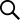В╣┤ьЁїЖ│авдг
Ж░ђЖ▓Е
ВЏљ ~ ВЏљ
Ж▓ђВЃЅВќ┤

HOME > >

# ВІаВёаВІЮьњѕВёИьіИ

ListQuery :
select P.*, C.C_name, if(sum(O.P_qty) is NULL, 0, sum(O.P_qty)) AS SUM_QTY from Plaza_product as P left join PRODUCT_FIX as F on P.P_uid = F.P_uid and F.DIR = '61' left join Company as C on P.P_comp_uid = C.C_uid left join Plaza_dir as D on P.P_dir like concat('%;', D.Dir, ';%') left join Plaza_order_cart as O on P.P_uid = O.P_uid where F.P_uid is NULL and P.P_show = 'show' and P.P_approval = 'Y' and P.P_status <> 'del' and C.C_approval = 'Y' and C.C_state = '' and D.Open = 'Y' and (P.P_dir like '%;61;%' or P.P_dir like '%;194;%' or P.P_dir like '%;195;%' or P.P_dir like '%;196;%' or P.P_dir like '%;206;%' ) group by P.P_uid order by P_regdate DESC, P_name asc, P_regdate limit -4, 20;
-->